## Example Questions

← Previous 1

### Example Question #1 : Acute / Obtuse Triangles

Two sides of an isosceles triangle are 20 and 30. What is the difference of the largest and the smallest possible perimeters?

10

15

0

30

10

Explanation:

The trick here is that we don't know which is the repeated side. Our possible triangles are therefore 20 + 20 + 30 = 70 or 30 + 30 + 20 = 80.  The difference is therefore 80 – 70 or 10.

### Example Question #1 : Isosceles Triangles

A triangle with two equal angles is called a(n) __________.

equilateral triangle

disjoint triangle

right triangle

Pythagoras triangle

isosceles triangle

isosceles triangle

Explanation:

An isoceles triangle is a triangle that has at least two congruent sides (and therefore, at least two congruent angles as well).

### Example Question #1 : How To Find An Angle In An Acute / Obtuse Isosceles Triangle

Triangle ABC has angle measures as follows: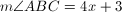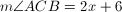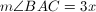What is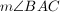?

19

90

79

57

44

57

Explanation:

The sum of the measures of the angles of a triangle is 180.

Thus we set up the equation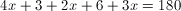After combining like terms and cancelling, we have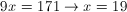Thus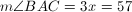### Example Question #2 : How To Find An Angle In An Acute / Obtuse Isosceles Triangle

The base angle of an isosceles triangle is five more than twice the vertex angle.  What is the base angle?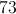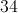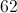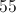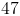Explanation:

Every triangle has 180 degrees.  An isosceles triangle has one vertex angle and two congruent base angles.

Let= the vertex angle and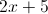= the base angle

So the equation to solve becomes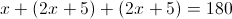Thus the vertex angle is 34 and the base angles are 73.

### Example Question #3 : How To Find An Angle In An Acute / Obtuse Isosceles Triangle

The base angle of an isosceles triangle is 15 less than three times the vertex angle.  What is the vertex angle?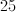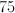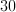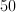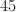Explanation:

Every triangle contains 180 degrees.  An isosceles triangle has one vertex angle and two congruent base angles.

Let= vertex angle and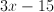= base angle

So the equation to solve becomes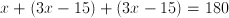.

### Example Question #1 : How To Find An Angle In An Acute / Obtuse Triangle

The base angle of an isosceles triangle is ten less than twice the vertex angle.  What is the vertex angle?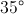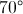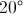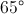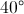Explanation:

Every triangle has 180 degrees.  An isosceles triangle has one vertex angle and two congruent base angles.

Let= vertex angle and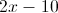= base angle

So the equation to solve becomes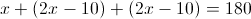So the vertex angle is 40 and the base angles is 70

### Example Question #1 : Acute / Obtuse Isosceles Triangles

The base angle of an isosceles triangle is 10 more than twice the vertex angle.  What is the vertex angle?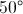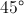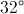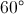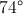Explanation:

Every triangle has 180 degrees.  An isosceles triangle has one vertex angle and two congruent base angles.

Let= the vertex angle and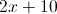= the base angle

So the equation to solve becomes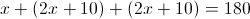The vertex angle is 32 degrees and the base angle is 74 degrees

### Example Question #42 : Triangles

In an isosceles triangle, the vertex angle is 15 less than the base angle.  What is the base angle?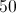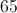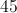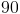Explanation:

Every triangle has 180 degrees.  An isosceles triangle has one vertex angle and two congruent base angles.

Let= base angle and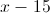= vertex angle

So the equation to solve becomes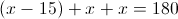Thus, 65 is the base angle and 50 is the vertex angle.

### Example Question #1 : How To Find An Angle In An Acute / Obtuse Isosceles Triangle

In an isosceles triangle the vertex angle is half the base angle.  What is the vertex angle?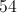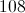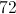Explanation:

Every triangle has 180 degrees.  An isosceles triangle has one vertex angle and two congruent base angles.

Let= base angle and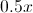= vertex angle

So the equation to solve becomes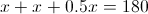, thus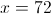is the base angle and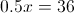is the vertex angle.

### Example Question #1 : Acute / Obtuse Isosceles Triangles

If the average (arithmetic mean) of two noncongruent angles of an isosceles triangle is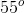, which of the following is the measure of one of the angles of the triangle?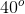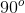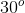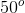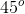Explanation:

Since the triangle is isosceles, we know that 2 of the angles (that sum up to 180) must be equal. The question states that the noncongruent angles average 55°, thus providing us with a system of two equations: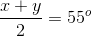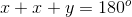Solving for x and y by substitution, we get x = 70° and y = 40° (which average out to 55°).

70 + 70 + 40 equals 180 also checks out.

Since 70° is not an answer choice for us, we know that the 40° must be one of the angles.

← Previous 1

### All SAT Math Resources